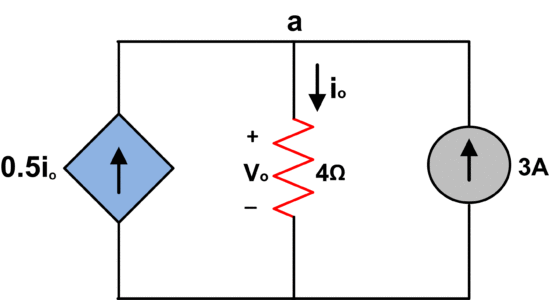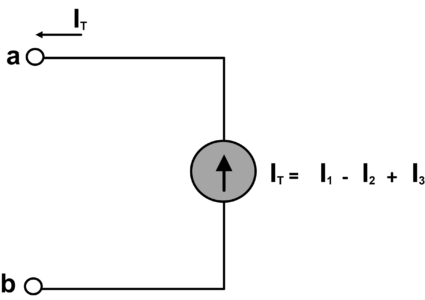Home / Basic Electrical / Kirchhoff’s Current law (KCL)

Kirchhoff’s Current law (KCL)

Want create site? Find Free WordPress Themes and plugins.

For a given circuit, a connection of two or more elements is called a NODE. The particular circuit shown in figure 1 depicts an example of a node.Figure.1: Circuit for Kirchhoff’s Current Law

We now present the Kirchhoff’s current law which is essentially the law of conservation of electric charge.

Kirchhoff’s Current LawAt any node of a circuit, the sum of the currents into the node is equal to the sum of the currents out of the node.

Since charge must be conserved and does not accumulate at a node, the amount of current flowing out of a node equals the amount flowing in at any instant. In other words, an electrical node acts like a junction of water pipes where the amount of water going out equals the amount coming in.

Specifically for the portion of the network shown in figure 1, by applying KCL we obtain the equation

${{i}_{3}}+{{i}_{4}}={{i}_{1}}+{{i}_{2}}$

An alternative, but equivalent, form of KCL can be obtained by considering currents directed into a node be positive in sense and currents directed out of a node to be negative in sense (or vice versa). Under this circumstance, the alternative form of KCL can be stated as follows:

KCL in an Alternate FormAt any node of a circuit, the currents algebraically sum to zero.

$\sum{i}=0$

Applying this form of KCL to the node in figure 1 and considering currents directed into be positive in sense, we get

${{i}_{1}}+{{i}_{2}}-{{i}_{3}}-{{i}_{4}}=0$

A close inspection of last two equations, however, reveals that they are the same.

Kirchhoff’s current Law Solved Example

Find I using KCLUsing KCL at node a,

$0.5{{i}_{0}}+3={{i}_{0}}$

${{i}_{0}}=6A$

Application of KCL

A very simple application of KCL is to combine current sources in parallel.Using KCL, we can convert above figure into single current source form.Current  I and  I are in the same direction as  IT total current, so we consider  I and  I as positive currents, while  I is in opposite direction of  I and  I3  so will be considered as negative current. So, the total resultant current  IT will be,

${{i}_{T}}={{i}_{1}}-{{i}_{2}}+{{i}_{3}}$

Did you find apk for android? You can find new Free Android Games and apps.